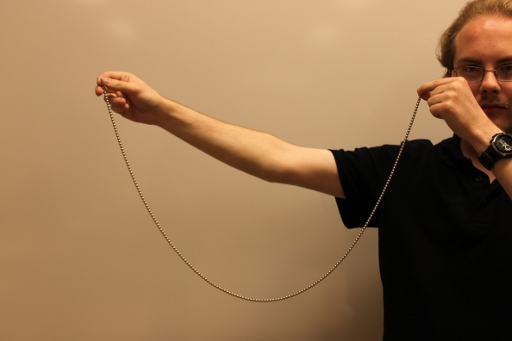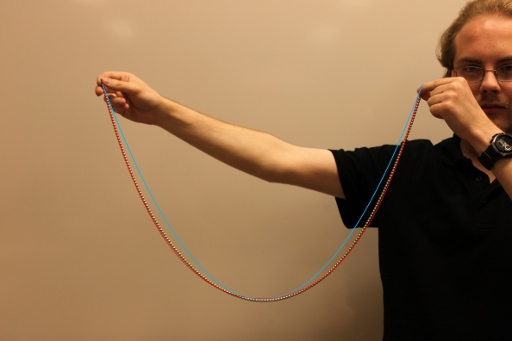$\newcommand{\sech}{\mathop{\rm sech}\nolimits} \newcommand{\csch}{\mathop{\rm csch}\nolimits} \newcommand{\R}{\mathbb{R}} \newcommand{\basis}{\underline{\mathbf{e}}}$
Current Page
Catenary

# Catenary

Object type: Plane curve

### Definition

The catenary is the graph of the hyperbolic cosine, or, more generally, the function $x \mapsto a \cosh {\left(\frac{x}{a}\right)}$ for some $a > 0$. In the image below, $a = 5$.### Curvature

The curvature function for the catenary (parameterised using $x$ as parameter) is the bump function $$\kappa(x) = \frac{1}{a} \text{sech}^2 {\left(\frac{x}{a}\right)}.$$

### Examples

An ideal chain (cable, rope, wire) placed in a (constant) gravitational field suspended at its ends will obtain the shape of the catenary. Below this is demonstrated by the author himself.Let us superimpose one parabola (blue) and one catenary (red):